## E8 sphere packing

Maple

According to Sphere Packing Solved in Higher Dimensions, the best way, i.e., most compact way, to pack spheres in dimensions 8 and 24 are done with the E8 lattice and Leech lattice, respectively. According to the Wikipedia article Leech lattice, the number of spheres that can be packed around any one sphere is 240 and 196,560 (!), respectively, the latter number of spheres counter-intuitively large. It inspired me to try to check that there is indeed room in these lattices for (at least) this number of spheres.

Starting with the E8 lattice: It is generated by the sum (over the integers) of all the 240 roots of E8. Following the prescription given in the subsection 'Construction' in the Wikipedia article E8 (mathematics), these roots may be constructed as follows:

`ROOTS := map(x -> Vector(x),[   # Coordinates all integers: 112 roots   combinat[permute]([+1,+1,0,0,0,0,0,0])[],   combinat[permute]([+1,-1,0,0,0,0,0,0])[],   combinat[permute]([-1,-1,0,0,0,0,0,0])[],   # Coordinates all half-integers: 128 roots   seq(combinat[permute]([      (+1/2)\$(  2*n),   # Even number of +1/2      (-1/2)\$(8-2*n)    # Even number of -1/2   ])[],n = 0..4)]):`

This Maple code gives a list of 240 eight-dimensional vectors. All these roots have the same length (the lattice thus being simply laced):

`convert(map(x -> Norm(x,2),ROOTS),set)[];`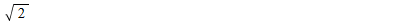If the distance between any pair of different roots is at least this length, then there will be room for 240 spheres of radius equal to this length around any one single sphere. And that is indeed the case:

`DIST_ROOTS := Matrix(nops(ROOTS)\$2,(i,j) ->   Norm(ROOTS[i] - ROOTS[j],2),shape = symmetric):min(convert(DIST_ROOTS,set) minus {0});`Using the above method for the Leech lattice will fail on grounds of hopeless performance, not the least because DIST_ROOTS will take ages to calculate, if at all possible. So any reader is welcome to weigh in with ideas on how to check the Leech lattice case.

PS: By the way, I was surprised to find that the three exceptional Lie algebras E6, E7, and E8 are seemingly not accessible through the Maple command SimpleLieAlgebraData, see its help page. Only the four infinite families A,B,C,D, as well as the two exceptional Lie algebras G2 and F4 are. Using Maple 17, I would like to know if that has been changed in Maple 17+, and if not, why not.

## Acceptable Maple solution?

by: Maple

In a recent post, the following inequality was proved with Maple: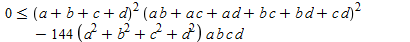(a,b,c,d >= 0).

Here is another direct proof attempt.

f:=(a+b+c+d)^2*(a*b+a*c+a*d+b*c+b*d+c*d)^2-144*(a^2+b^2+c^2+d^2)*a*b*c*d:
g:=expand(eval(f,d=1)):
s:=minimize(g, [a=0..infinity,b=0..infinity,c=0..infinity]):
length(s);   # huge
304856
map(evalf@evalf,s);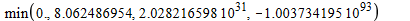map(evalf@evalf,s);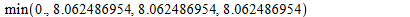So, Maple returns the expression

min(0,r1,r2,r3)

where r1,r2,r3 are huge expressions containing RootOfs. In order to evaluate them, several hundreds of digits are needed.
The solution seems to be correct, but the question is: may we (mathematically) accept it? What do you think?

## Inequality proved with Maple. II

Maple

How to prove the inequality, assuming that the  variables are nonnegative? That hard question  was asked by arqady in dxdy and answered  by himself  in a complicated way. Maple proves the inequality by the LagrangeMultipliers command which is strong. I think these calculations cannot be done by hand at all. Without loss of generality one may assume. Then

restart:with(Student[MultivariateCalculus]):

ans := [LagrangeMultipliers((a+b+c+d)*(a*b+a*c+a*d+b*c+b*d+c*d)-12*sqrt((a^2+b^2+c^2+d^2)*a*b*c*d), [a+b+c+d-1], [a, b, c, d], output = detailed)]:

We have to remove complex solutions by
ans1:=remove(c -> has(evalf(c), I),ans):

The next big output is  only partly seen in the post (look in the attached file for the whole one).

 [[a = 1/6, b = 1/2, c = 1/6, d = 1/6, lambda = 0, -12*sqrt((a^2+b^2+c^2+d^2)*a*b*c*d)+(b+c+d)*a^2+(b^2+(3*c+3*d)*b+c^2+3*c*d+d^2)*a+(d+c)*b^2+(c^2+3*c*d+d^2)*b+c^2*d+c*d^2 = 0],[a = 1/4, b = 1/4, c = 1/4, d = 1/4, lambda = 0, -12*sqrt((a^2+b^2+c^2+d^2)*a*b*c*d)+(b+c+d)*a^2+(b^2+(3*c+3*d)*b+c^2+3*c*d+d^2)*a+(d+c)*b^2+(c^2+3*c*d+d^2)*b+c^2*d+c*d^2 = 0],[a = 13/72-(1/216)*sqrt(3)*sqrt((2*(11548+108*sqrt(13397))^(2/3)+11*(11548+108*sqrt(13397))^(1/3)-568)/(11548+108*sqrt(13397))^(1/3))+(1/216)*sqrt(3)*sqrt(2)*sqrt((11*(11548+108*sqrt(13397))^(1/3)-(11548+108*sqrt(13397))^(2/3)+284)/(11548+108*sqrt(13397))^(1/3)+273*sqrt(3)/sqrt((2*(11548+108*sqrt(13397))^(2/3)+11*(11548+108*sqrt(13397))^(1/3)-568)/(11548+108*sqrt(13397))^(1/3))), b = 11/24+(1/72)*sqrt(3)*sqrt((2*(11548+108*sqrt(13397))^(2/3)+11*(11548+108*sqrt(13397))^(1/3)-568)/(11548+108*sqrt(13397))^(1/3))-(1/72)*sqrt(3)*sqrt(2)*sqrt((11*(11548+108*sqrt(13397))^(1/3)-(11548+108*sqrt(13397))^(2/3)+284)/(11548+108*sqrt(13397))^(1/3)+273*sqrt(3)/sqrt((2*(11548+108*sqrt(13397))^(2/3)+11*(11548+108*sqrt(13397))^(1/3)-568)/(11548+108*sqrt(13397))^(1/3))), c = 13/72-(1/216)*sqrt(3)*sqrt((2*(11548+108*sqrt(13397))^(2/3)+11*(11548+108*sqrt(13397))^(1/3)-568)/(11548+108*sqrt(13397))^(1/3))+(1/216)*sqrt(3)*sqrt(2)*sqrt((11*(11548+108*sqrt(13397))^(1/3)-(11548+108*sqrt(13397))^(2/3)+284)/(11548+108*sqrt(13397))^(1/3)+273*sqrt(3)/sqrt((2*(11548+108*sqrt(13397))^(2/3)+11*(11548+108*sqrt(13397))^(1/3)-568)/(11548+108*sqrt(13397))^(1/3))), d = 13/72-(1/216)*sqrt(3)*sqrt((2*(11548+108*sqrt(13397))^(2/3)+11*(11548+108*sqrt(13397))^(1/3)-568)/(11548+108*sqrt(13397))^(1/3))+(1/216)*sqrt(3)*sqrt(2)*sqrt((11*(11548+108*sqrt(13397))^(1/3)-(11548+108*sqrt(13397))^(2/3)+284)/(11548+108*sqrt(13397))^(1/3)+273*sqrt(3)/sqrt((2*(11548+108*sqrt(13397))^(2/3)+11*(11548+108*sqrt(13397))^(1/3)-568)/(11548+108*sqrt(13397))^(1/3))), lambda = -(5/36)*(sqrt(2)*(sqrt(3)*(sqrt(13397)-(71/27)*(11548+108*sqrt(13397))^(1/3)-(103/540)*(11548+108*sqrt(13397))^(2/3)+2887/27)*sqrt((2*(11548+108*sqrt(13397))^(2/3)+11*(11548+108*sqrt(13397))^(1/3)-568)/(11548+108*sqrt(13397))^(1/3))-15*sqrt(13397)+(355/9)*(11548+108*sqrt(13397))^(1/3)+(109/36)*(11548+108*sqrt(13397))^(2/3)-14435/9)*sqrt((11*(11548+108*sqrt(13397))^(1/3)-(11548+108*sqrt(13397))^(2/3)+284)/(11548+108*sqrt(13397))^(1/3)+273*sqrt(3)/sqrt((2*(11548+108*sqrt(13397))^(2/3)+11*(11548+108*sqrt(13397))^(1/3)-568)/(11548+108*sqrt(13397))^(1/3)))-(133/15)*(11548+108*sqrt(13397))^(2/3)*sqrt((2*(11548+108*sqrt(13397))^(2/3)+11*(11548+108*sqrt(13397))^(1/3)-568)/(11548+108*sqrt(13397))^(1/3))+(2*((sqrt(13397)+2374/45)*(11548+108*sqrt(13397))^(1/3)+(103/5)*sqrt(13397)+(449/90)*(11548+108*sqrt(13397))^(2/3)+132727/45))*sqrt(3))/((11548+108*sqrt(13397))^(2/3)*sqrt((2*(11548+108*sqrt(13397))^(2/3)+11*(11548+108*sqrt(13397))^(1/3)-568)/(11548+108*sqrt(13397))^(1/3))), -12*sqrt((a^2+b^2+c^2+d^2)*a*b*c*d)+(b+c+d)*a^2+(b^2+(3*c+3*d)*b+c^2+3*c*d+d^2)*a+(d+c)*b^2+(c^2+3*c*d+d^2)*b+c^2*d+c*d^2 = -(13/46656)*(((2/13)*sqrt((11*(11548+108*sqrt(13397))^(1/3)-(11548+108*sqrt(13397))^(2/3)+284)/(11548+108*sqrt(13397))^(1/3)+273*sqrt(3)/sqrt((2*(11548+108*sqrt(13397))^(2/3)+11*(11548+108*sqrt(13397))^(1/3)-568)/(11548+108*sqrt(13397))^(1/3)))*(11548+108*sqrt(13397))^(1/3)*sqrt((2*(11548+108*sqrt(13397))^(2/3)+11*(11548+108*sqrt(13397))^(1/3)-568)/(11548+108*sqrt(13397))^(1/3))+sqrt(2)*(sqrt(3)*(11548+108*sqrt(13397))^(1/3)*sqrt((2*(11548+108*sqrt(13397))^(2/3)+11*(11548+108*sqrt(13397))^(1/3)-568)/(11548+108*sqrt(13397))^(1/3))-(11/13)*(11548+108*sqrt(13397))^(1/3)-(2/13)*(11548+108*sqrt(13397))^(2/3)+568/13))*sqrt(5)*sqrt((sqrt(3)*sqrt(2)*sqrt((11*(11548+108*sqrt(13397))^(1/3)-(11548+108*sqrt(13397))^(2/3)+284)/(11548+108*sqrt(13397))^(1/3)+273*sqrt(3)/sqrt((2*(11548+108*sqrt(13397))^(2/3)+11*(11548+108*sqrt(13397))^(1/3)-568)/(11548+108*sqrt(13397))^(1/3)))-sqrt(3)*sqrt((2*(11548+108*sqrt(13397))^(2/3)+11*(11548+108*sqrt(13397))^(1/3)-568)/(11548+108*sqrt(13397))^(1/3))-33)*(sqrt(3)*sqrt(2)*sqrt((11*(11548+108*sqrt(13397))^(1/3)-(11548+108*sqrt(13397))^(2/3)+284)/(11548+108*sqrt(13397))^(1/3)+273*sqrt(3)/sqrt((2*(11548+108*sqrt(13397))^(2/3)+11*(11548+108*sqrt(13397))^(1/3)-568)/(11548+108*sqrt(13397))^(1/3)))-sqrt(3)*sqrt((2*(11548+108*sqrt(13397))^(2/3)+11*(11548+108*sqrt(13397))^(1/3)-568)/(11548+108*sqrt(13397))^(1/3))+39)*(sqrt(2)*(sqrt(3)*(11548+108*sqrt(13397))^(1/3)*sqrt((2*(11548+108*sqrt(13397))^(2/3)+11*(11548+108*sqrt(13397))^(1/3)-568)/(11548+108*sqrt(13397))^(1/3))+(11/5)*(11548+108*sqrt(13397))^(1/3)+(2/5)*(11548+108*sqrt(13397))^(2/3)-568/5)*sqrt((11*(11548+108*sqrt(13397))^(1/3)-(11548+108*sqrt(13397))^(2/3)+284)/(11548+108*sqrt(13397))^(1/3)+273*sqrt(3)/sqrt((2*(11548+108*sqrt(13397))^(2/3)+11*(11548+108*sqrt(13397))^(1/3)-568)/(11548+108*sqrt(13397))^(1/3)))-(216/5)*(11548+108*sqrt(13397))^(1/3)*sqrt((2*(11548+108*sqrt(13397))^(2/3)+11*(11548+108*sqrt(13397))^(1/3)-568)/(11548+108*sqrt(13397))^(1/3))-(328/5*((11548+108*sqrt(13397))^(1/3)+(5/164)*(11548+108*sqrt(13397))^(2/3)-355/41))*sqrt(3))/((11548+108*sqrt(13397))^(1/3)*sqrt((2*(11548+108*sqrt(13397))^(2/3)+11*(11548+108*sqrt(13397))^(1/3)-568)/(11548+108*sqrt(13397))^(1/3))))-(180/13)*sqrt(2)*(sqrt(3)*(11548+108*sqrt(13397))^(1/3)*sqrt((2*(11548+108*sqrt(13397))^(2/3)+11*(11548+108*sqrt(13397))^(1/3)-568)/(11548+108*sqrt(13397))^(1/3))+(11/5)*(11548+108*sqrt(13397))^(1/3)+(2/5)*(11548+108*sqrt(13397))^(2/3)-568/5)*sqrt((11*(11548+108*sqrt(13397))^(1/3)-(11548+108*sqrt(13397))^(2/3)+284)/(11548+108*sqrt(13397))^(1/3)+273*sqrt(3)/sqrt((2*(11548+108*sqrt(13397))^(2/3)+11*(11548+108*sqrt(13397))^(1/3)-568)/(11548+108*sqrt(13397))^(1/3)))-(15552/13)*(11548+108*sqrt(13397))^(1/3)*sqrt((2*(11548+108*sqrt(13397))^(2/3)+11*(11548+108*sqrt(13397))^(1/3)-568)/(11548+108*sqrt(13397))^(1/3))+(11808/13*((11548+108*sqrt(13397))^(1/3)+(5/164)*(11548+108*sqrt(13397))^(2/3)-355/41))*sqrt(3))/((11548+108*sqrt(13397))^(1/3)*sqrt((2*(11548+108*sqrt(13397))^(2/3)+11*(11548+108*sqrt(13397))^(1/3)-568)/(11548+108*sqrt(13397))^(1/3)))] (1)

evalf(ans2);(2)

Indeed, the minimum value of the target function is exactly 0. Quod erat demonstrantum.## Transforming Functions to Names: Further explaining...

by: Maple

A wealth of knowledge is on display in MaplePrimes as our contributors share their expertise and step up to answer others’ queries. This post picks out one such response and further elucidates the answers to the posted question. I hope these explanations appeal to those of our readers who might not be familiar with the techniques embedded in the original responses.

The Question: Transforming functions to names

Bendesarts wanted to know how to make programmatic changes to characters in a list. He wrote:

I have this list :

T:=[alpha(t),beta(t)]

I would like to create this list automatically:

Tmod:=[alpha_,beta_]

In other words, how can I remove the 3 characters "(t)" and replace it by "_"

Do you have ideas to do so ?

Thanks a lot for your help

Joe Riel provided a complete answer that had three different approaches. He wrote:

Map, over the list, a procedure that extracts the name of the function and catenates an underscore to it. The function name is extracted via the op procedure, e.g. op(0,f(a,b,c)) evaluates to f. Thus

map(f->cat(op(0,f),_),T);

Note that this fails if the name of a function is indexed, e.g. f(a). No error is generated but the catenation doesn't evaluate to a symbol. Presumably that isn't an issue here.  One way to handle that case is to first convert the indexed name to a symbol, then catenate the underscore.  So a more robust version is

map(f->cat(convert(op(0,f),'symbol'),_),T);

However, if you are actually dealing with indexed names you might want a different result. Another way to do the conversion, and combine it with the catenation, is to use nprintf, which generates a name (symbol). Thus

map(f -> nprintf("%a_", op(0,f)),T);

Let’s discuss each approach by understanding the definitions and functionalities of the commands used.

The map command, map(fcn, expr, arg1, ..., argN) applies a procedure or name, fcn, to the operands or elements of an expression, expr. The result of a call to map is a copy of expr with the ith operand of expr replaced by the result of applying fcn to the ith operand.  This concept is easier to grasp by looking at a few examples related to the usage of map in this question.

Example 1.  map(x-> x2,x+y)         returns     x2+y2

Example 2. map(a -> a-b, sin(x))    returns     sin(x-b)

The cat function, cat(a,b,c,…), is commonly used to concatenate (or join) string and names together. This function’s parameters: a,b,c…, can be any expressions.

Example 1. cat(a,2)                      returns     a2

Example 2.  cat(“a”,3,sin(x))          returns    “a3sin(x)”

The op function, op(i..j,e), extracts operands from an expression. The parameters i and j are the integers indicating positions of the operands and e is the expression. For functions, as in this example, op(0,e) is the name of the function.

Example 1.  op(0,alpha(t))            returns   the symbol alpha

Example 2.  op(0, sin(x))              returns    sin

Now analyzing Joe Riel's code will be easier.

1. map(f->cat(op(0,f),_),T);

In this approach Joe is extracting the name of the functions, alpha and beta, and then concatenating it to the underscore symbol. Then using the mapping function he applies the previous procedure to the list T.

1. map(f->cat(convert(op(0,f),'symbol'),_),T);

This approach is a lot similar to the previous one, but he added the convert function in case the function inside of map was indexed. Convert(expr, form, arg3,..), is used to change an expression from one form to another. In this example op(0,f) has been changed from type name to type symbol.

1. map(f -> nprintf("%a_", op(0,f)),T);

Again this is a similar approach but it uses nprintf. This command, nprintf(fmt,x1,..xn), is based on a C standard library command of the same name. It uses the format specifications in the fmt string to format and writes the expression into a Maple symbol, which is returned. In this example the format specified is the algebraic format “%a”.

This blog was written by Maplesoft’s intern Pia under the supervision of Dr. Robert Lopez. We both hope that you find this useful. If there is a particular question on MaplePrimes that you would like further explained, please let us know.

## The mechanism of transport of the material. Animation...

by: Maple 15

The mechanism of transport of the material of the sewing machine M 1022 class: mathematical animation.   BELORUS.mw## Inequality proved with Maple

Maple

How to prove the inequalityprovided,? That problem was posed  by Israeli mathematician nicked by himself as arqady in Russian math forum and was not answered there.I know how to prove that with Maple and don't know how to prove that without Maple. Neithernorwork here. The difficulty consists in the nonlinearity both the target function and the main constraint. The first step is to linearize the main constraint and the second step is to reduce the number of variables to one.

restart; A := eval(x^(4*y)+y^(4*x), [x = sqrt(u), y = sqrt(v)]);(1)

B := expand(A);(2)

C := eval(B, u = 2-v);(3)

It is more or less clear that the plot of F is symmetric wrt  the straight line v=1. This motivates the following change of variable  to obtain an even function.

F := simplify(expand(eval(C, v = z+1)), symbolic, power);(4)The plots suggest the only maximim of F at z=0 and its concavity.

Student[Calculus1]:-FunctionPlot(F, z = -1 .. 1);Student[Calculus1]:-FunctionPlot(diff(F, z, z), z = -1 .. 1);As usually, numeric global solvers cannot prove certain inequalities. However, the GlobalSearch command of the DirectSearch package indicates the only local maximum of  F and F''.Digits := 25; DirectSearch:-GlobalSearch(F, {z = -1 .. 1}, maximize, solutions = 3, tolerances = 10^(-15)); DirectSearch:-GlobalSearch(diff(F, z, z), {z = -1 .. 1}, maximize, solutions = 3, tolerances = 10^(-15));(5)

The series command confirms a local maximum of F at z=0.

series(F, z, 6);(6)

The extrema command indicates only the value of F at a critical point, not outputting its position.

extrema(F, z); extrema(F, z, 's');(7)

solve(F = 2);(8)

DirectSearch:-SolveEquations(F = 2, {z = -1 .. 1}, AllSolutions, solutions = 3);(9)

DirectSearch:-SolveEquations(F = 2, {z = -1 .. 1}, AllSolutions, solutions = 3, assume = integer);(10)PS. I see my proof needs an additional explanation. The DirectSearch command establishes the only both local and global  maximum of F is located at z= -1.98*10^(-13) up to default error 10^(-9). After that  the series command confirms a local maximum at z=0. Combining these, one draws the conclusion that the global maximum is placed exactly at z=0 and equals 2. In order to confirm that the only real root of F=2 at z=0  is found approximately and exactly by the DirectSearch.

## Sparse Matrix Products in Maple

by: Maple

I thought I would share some code for computing sparse matrix products in Maple.  For floating point matrices this is done quickly, but for algebraic datatypes there is a performance problem with the builtin routines, as noted in http://www.mapleprimes.com/questions/205739-How-Do-I-Improve-The-Performance-Of

The code is fairly straightforward in that it uses op(1,A) to extract the dimensions and op(2,A) to extract the non-zero elements of a Matrix or Vector, and then loops over those elements.  I included a sparse map function for cases where you want to map a function (like expand) over non-zero elements only.

`# sparse matrix vector productspmv := proc(A::Matrix,V::Vector)local m,n,Ae,Ve,Vi,R,e;  n, m := op(1,A);  if op(1,V) <> m then error "incompatible dimensions"; end if;  Ae := op(2,A);  Ve := op(2,V);  Vi := map2(op,1,Ve);  R := Vector(n, storage=sparse);  for e in Ae do    n, m := op(1,e);    if member(m, Vi) then R[n] := R[n] + A[n,m]*V[m]; end if;  end do;  return R;end proc:`
`# sparse matrix productspmm := proc(A::Matrix, B::Matrix)local m,n,Ae,Be,Bi,R,l,e,i;  n, m := op(1,A);  i, l := op(1,B);  if i <> m then error "incompatible dimensions"; end if;  Ae := op(2,A);  Be := op(2,B);  R := Matrix(n,l,storage=sparse);  for i from 1 to l do    Bi, Be := selectremove(type, Be, (anything,i)=anything);    Bi := map2(op,[1,1],Bi);    for e in Ae do      n, m := op(1,e);      if member(m, Bi) then R[n,i] := R[n,i] + A[n,m]*B[m,i]; end if;    end do;  end do;  return R;end proc:`
`# sparse mapsmap := proc(f, A::{Matrix,Vector})local B, Ae, e;  if A::Vector then    B := Vector(op(1,A),storage=sparse):  else    B := Matrix(op(1,A),storage=sparse):  end if;  Ae := op(2,A);  for e in Ae do    B[op(1,e)] := f(op(2,e),args[3..nargs]);  end do;  return B;end proc:`

As for how it performs, here is a demo inspired by the original post.

`n := 674;k := 6;`
`A := Matrix(n,n,storage=sparse):for i to n do  for j to k do    A[i,irem(rand(),n)+1] := randpoly(x):  end do:end do:`
`V := Vector(n):for i to k do  V[irem(rand(),n)+1] := randpoly(x):end do:`
`C := CodeTools:-Usage( spmv(A,V) ):  # 7ms, 25x fasterCodeTools:-Usage( A.V ):  # 174 ms`
`B := Matrix(n,n,storage=sparse):for i to n do  for j to k do    B[i,irem(rand(),n)+1] := randpoly(x):  end do:end do:`
`C := CodeTools:-Usage( spmm(A,B) ):  # 2.74 sec, 50x fasterCodeTools:-Usage( A.B ):  # 2.44 min`
`# expand and collect like termsC := CodeTools:-Usage( smap(expand, C) ):`

## Camera Profiler

by: Maple 18

CameraProfiler.mw

D700_Profile.xlsx

This Application uses the xrite color checker to obtain Forward and Color Matrices as defined by Adobe.

It compares the camera gamut based on the above matrices to the 1931 Standard Observer using LEDs.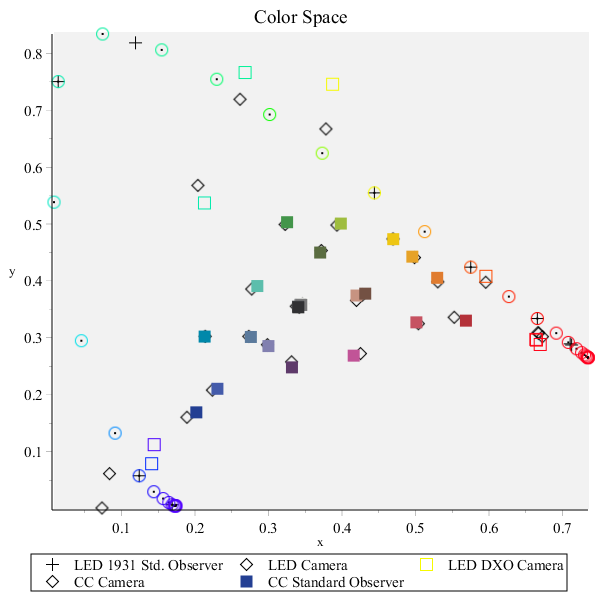## Rolling without slipping animation

by: Maple 15

Continuation.
One way to get rolling without slipping animation in 3d. The trajectory and circle are divided into segments of equal length. In the next segment of the trajectory we construct circle, taking into account the fact that it turned on one segment. Rolling sphere or cylinder can be simulated, if we take plottools templates of the same radius, and replace them on the site of our circle.

ROLLING_WITHOUT_3d.mw## How to sketch a straight line in three-dimensional...

by: Maple

A wealth of knowledge is on display in MaplePrimes as our contributors share their expertise and step up to answer others’ queries. This post picks out one such response and further elucidates the answers to the posted question. I hope these explanations appeal to those of our readers who might not be familiar with the techniques embedded in the original responses.

The Question: How to sketch a line in space?

vahid65 wanted to know how to sketch the line with equation (x-2)/3 = (y-1)/4 = (z-3)/3 in a three-dimensional space.

This question was answered using two different approaches that we will discuss.

The first approach, given by Preben Alsholm, suggested using these commands: solve, subs, and plots:-spacecurve.

Preben provided the following lines of code:

1. {(x-2)/3 , (y-1)/4 , (z-3)/3} =~t;
2. solve(%,{x,y,z});
3. L:=subs(%,[x,y,z]);
4. plots:-spacecurve(L,t=-5..5,thickness=3,color=red);

The first line sets the three expressions equal to t using the element wise operator =~.  This distributes the operation of equality over the elements of the set of expressions, forming a set of equations.

Result-1: {(x-2)/3=t, (y-1)/4=t, (z-3)/3=t}

The second line invokes the solve command. The solve command, solve(equations, variables), solves one or more equations or inequalities for their unknowns. So in this line, this command was used to solve each expression for its corresponding unknown.

Result-2: {(x= 2+3t, y=1+4t, z= 3+3t}

You may have noticed that the % symbol is used within the command. This symbol is referring to the equation label that was created chronologically last.

The third line uses the subs command. The subs command, subs(x=a,expr), substitutes “a” for “x” in the expression expr. In this case, “a” is the reference to the equation label referenced by (%) and expr is the sequence of variables x,y,z. The square brackets around expr forms a list, and the replacement imposed by the subs command replaces each of the three names x, y, and z with their equivalents in the set of equations returned by the solve command.

Result-3: [2+3t,1+4t, 3+3t ]

Finally the last line uses the plots:-spacecurve function.  This function, spacecurve(sc,r,opts),  graphs a parametrically defined curve in three-dimensional Cartesian space.

In this example the first parameter, sc, is replaced by L since L has the properties of a list. The parameter  r is an equation containing the parameter name and the parameter range; here it is the equation t=-5..5. Last but not least, the opts parameter (which is optional) gives the user the opportunity to specify the optional properties of the graph, properties such as thickness and color of the space curve.

Result 4:Another contributer, Carl Love, suggested that these commands could be combined using the zip function, zip( f, u, v), which is an unusual application of it. The zip command applies elementwise to corresponding members of the two lists u and v, the binary function f, creating a new list r, whose members are f(uk,vk).

Carl provided the following code:

plots:-spacecurve(  zip(solve, [(x-2)/3 , (y-1)/4 , (z-3)/3] =~ t, [x,y,z]),  t= -5..5, thickness= 3, color= red, axes= normal);

In this case zip is applying solve to u, a list of equations, and v, a list of variables. The equations in u are those in Result-1. The zip function returns the solution of each equation for the indicated variable, that is, the new list r is the list in Result-3. This list is the first argument of plots:-spacecurve. Finally, the parameter range and opts are up to the user’s preferences.

A second approach was suggested by Kitonum. The difference between this approach and the first one is that here we are not using the solve command. Instead, we are treating the line as an intersection of two planes.

Kitonum's code is as follows:

1. L := [(x-2)*(1/3), (y-1)*(1/4), (z-3)*(1/3)]:
2. plots[intersectplot](L-L, L-L, x = -4 .. 4, y = -4 .. 4, z = -4 .. 4, linestyle = 1, thickness = 3, axes = normal, orientation = [60, 75], view = [-1 .. 3, -4 .. 2, -1 .. 4]);

The first statement initializes a list L that contains all the desired expressions in the given symmetric form of the line.

In the second statement Kionum used the plots[intersectplot] command. This command graphs, in three-dimensional Cartesian space, the intersection of a pair of surfaces. This example uses the calling sequence: intersectplot(expr1, expr2, x=a..b, y=c..d, z= e..f, options)

What are expr1 and expr2 used in Kitonum’s solution? The pairwise differences of L and L, and L and L are planes, the intersection of which is the desired line in space.

expr1 = L – L = (x-2)*(1/3)- (y-1)*(1/4)

expr2= L - L = (y-1)*(1/4)-(z-3)*(1/3)

The variables x,y, and z are the coordinate names and define the axes, whereas a, b, c, d, e, f define the ranges. In this case they were taken as -4 or 4.

In the options parameter Kitonum used linestyle, thickness, axes, orientation, and  view. The help page “plot3d,option” details these and other options that apply.

This blog was written by our intern Pia under the supervision of Dr. Robert Lopez. We both hope that you find this useful. If there is a particular question on MaplePrimes that you would like further explained, please let us know.

## Spiral around the curve

by: Maple 15

Spiral (equidistant) around the curve.  In this case, a spiral around the spiral.
So without any sense.
spiral_around_curve.mw

If we re-save the animation with the program Easy GIF Animator, its size is reduced by about 10 times, and sometimes much more.## New and improved integration results in Maple...

by: Maple 2016

The attached worksheet shows a small selection of new and improved results in integration for Maple 2016. Note that integration is a vast topic, so there will always be more improvements that can be made, but be sure that we are working on them.

A selection of new and improved integration results for Maple 2016

Indefinite integrals:

 > int(sqrt(1+sqrt(z-1)), z);(1.1)
 > int(arctan((-1+sec(x))^(1/2))*sin(x), x);(1.2)
 > int(((1+exp(I*x))^2+(1+exp(-I*x))^2)/(1-2*c*cos(x)+c^2), x);(1.3)
 > int(x^4/arccos(x)^(3/2),x);(1.4)

Definite integrals:

 > int(arcsin(sin(z)), z=0..1);(1.5)
 > int(sqrt(1 - sqrt(1+z)), z=0..1);(1.6)
 > int(z/(exp(2*z)+4*exp(z)+10),z = 0 .. infinity);(1.7)
 > simplify(int(sinh(a*abs(x-y)), y=0..c, 'method'='FTOC'));(1.8)
 > int(ln(x+y)/(x^2+y), [x=0..infinity, y=0..infinity]);(1.9)

Definite integrals with assumptions on the parameters:

 > int(x^(-ln(x)),x=0..b) assuming b > 0;(1.10)
 > int(exp(-z)*exp(-I*n*z)*cos(n*z),z = -infinity .. infinity) assuming n::integer;(1.11)

Integral of symbolic integer powers of sin(x) or cos(x):

 > int(sin(x)^n,x) assuming n::integer;(1.12)
 > int(cos(x)^n,x) assuming n::negint;(1.13)
 > int(cos(x)^n,x) assuming n::posint;(1.14)

 > int(sqrt(1+sqrt(x)), x);(2.1)
 > int(sqrt(1+sqrt(1+z)), z= 0..1);(2.2)
 > int(signum(z^k)*exp(-z^2), z=-infinity..infinity) assuming k::real;(2.3)
 > int(2*abs(sin(x*p)*sin(x)), x = 0 .. Pi) assuming p> 1;(2.4)
 > int(1/(x^4-x+1), x = 0 .. infinity);(2.5)

In Maple 2016, this multiple integral is computed over 3 times faster than it was in Maple 2015.

 > int(exp(abs(x1-x2))*exp(abs(x1-x3))*exp(abs(x3-x4))*exp(abs(x4-x2)), [x1=0..R, x2=0..R, x3=0..R, x4=0..R], AllSolutions) assuming R>0;(2.6)

Austin Roche
Mathematical Software, Maplesoft

## Variable Identification: Further explaining a...

by: Maple

A wealth of knowledge is on display in MaplePrimes as our contributors share their expertise and step up to answer others’ queries. This post picks out one such response and further elucidates the answers to the posted question. I hope these explanations appeal to those of our readers who might not be familiar with the techniques embedded in the original responses.

The Question: Variable Identification

Don Carota wanted to know the best approach for finding variables that are not assigned to a value within an equation. He wrote:

I got a set of equations to solve, like this one:

eq:=W[1,0]*(a*HRa[1,0]+b*ga[1,0]+c)=d*SR[1,1]/grid

a,b,c,d are numbers, like 2.0458 and so on.

When I want to solve the set, I need to tell Maple the command solve:

solve( {seq(eq[i],i=1..N)},{variables});  (N is an integer of course)

To set the variables, one must check each equation to write: {W[1,0],HRa[1,0],ga[1,0]...} and so on.

I know that I can use the command is(variable,assignable) to check if a variable has not a value assigned already and, according to true/false I can construct the set {variables} and solve the set of equations.

That´s an easy solution if I need to check variables under a certain pattern, like: X, X, X since I can create a loop and check them one after the other. But my problem is that I have different names for variables or that variables change to assignable from assigned according to other conditions, so I can never be sure if W[1,0] is going to be a variable to compute in all steps instead of SR[1,1].

for example:

if a>3 then
SR[1,1]:=1/2;
else
W[1,0]:=300:
end if;

So, when I need to type solve, the {variables} part is different according to each case. Is there any command that allows me to insert an expression and Maple can return me the variables or parameters in the expression that are not numeric already?

(note that the link added to the is command above was added by me, not the original author)

dharr and Carl Love provided solutions that use the indets command.

The code provided by dharr is as follow:

1. indets(eq,name);

Result: gives the indeterminates: {a, b, c, d, HRa[1, 0], SR[1, 1], W[1, 0], ga[1, 0]}

The code provided by Carl Love is as follows:

1.       indets(eq, assignable(name));

or, doing all equations at once,

2.       indets({entries(eq, nolist)}, assignable(name));

Further Explaining the indets and type commands.

Both dharr and Carl Love provided an answer that used the indets command. In essence the indets command used in this example contains two arguments: indets(expr, typename). Expr is a rational expression that only uses the operations such as addition, subtraction, division, and multiplication. Typename is a parameter used when the desired return is a set containing all subexpressions in expr that are of type typename.

Carl Love used the assignable(name) argument  for the typename parameter in order to return all the variables that can be assigned a value, excluding constants such as Pi that are also considered names. Indeed, assignable is a type and can be used without an additional argument. For example, the command indets(x+f(x)+y=1, assignable) returns {x,y,f(x)} because all three symbols can be assigned values. However, indets(x+f(x)+y=1, assignable(name)) returns just {x,y} because f(x) is of type function, not of type name. Similarly, indets(x+y=Pi, assignable) would return just {x,y} because Pi is not considered to be something that can be assigned to.

Carl’s second command used ({entries(eq, nolist)} as the expr parameter. In this version, eq is the table whose members are the individual equations. Remember, the syntax x creates a table whose name is x, and whose entry is the object assigned to x. The entries(t) function returns a sequence of the table members, each member being placed in list brackets. By including the option nolist, the return is then a sequence of table members without list brackets.

Finally, note that different programmers will use different approaches to finding “indeterminants” in expressions. Dr. Lopez pointed out that some years ago he asked three different programmers about extracting the “assignable names” in an expression such as q:=x+Pi+cos(a). The naive indets(q) returns {a,x,cos(a)}, whereas indets(q,name) returns {Pi,a,x}. However, select(type,indets(q),name) returns {a,x}, as does indets(q,And(symbol,Not(constant))).

Don Carota’s question is able to showcase some of the different types that are within Maple’s platform. Therefore, it is important to go over what the type-checking function is and what it does. In many contexts, it is not necessary to know the exact value of an expression; instead it is enough to know if the value belongs to a group of expressions that have similarities. Such groups are knows as types.

Maple’s engine uses the type function in every single procedure to direct and maintain the flow of control in algorithms and to decide if the user’s input is valid. There are about 240 different types that Maple recognizes including: prime, string, symbol, list, etc.  Let’s see some examples of how the function works using elements from this question.

Type has two parameters: an expression e, and a valid type expression t. To check that the output of the entries(eq,nolist) is indeed not a list, the type command can be used as follows:As expected, the last command returns false! If you want to learn more about the type and indets commands you can visit their corresponding help pages: ?type, ?indets.

This blog was written by Maplesoft’s intern Pia under the supervision of Dr. Robert Lopez. We both hope that you find this useful. If there is a particular question on MaplePrimes that you would like further explained, please let us know.

## Both sides of the polygon in 3d

by: Maple 15

polygon_2_color.mw

Imitation coloring both sides of the polygon in 3d.  We  build a new polygon in parallel with our polygon on a very short distance t. (We need any three points on the polygon plane, do not lie on a straight line.) This place in the program is highlighted in blue.

Paint the polygons are in different colors.## New Thermal Engineering Applications on Application...

by: Maple 2016

Valery Ochkov and Volodymyr Voloshchuk have developed a series of thermal engineering applications in Maple 2016. The applications explore steam turbine power generation and refrigeration cycles, and use the ThermophysicalData package for fluid properties.

Their work can be found at the following locations on the Application Center.

I especially like

• this application, which optimizes the extraction pressures of a steam turbine to maximize its efficiency,
• and this application, which plots the state of a two-stage refrigeration cycle on a pressure-enthalpy chart.﻿